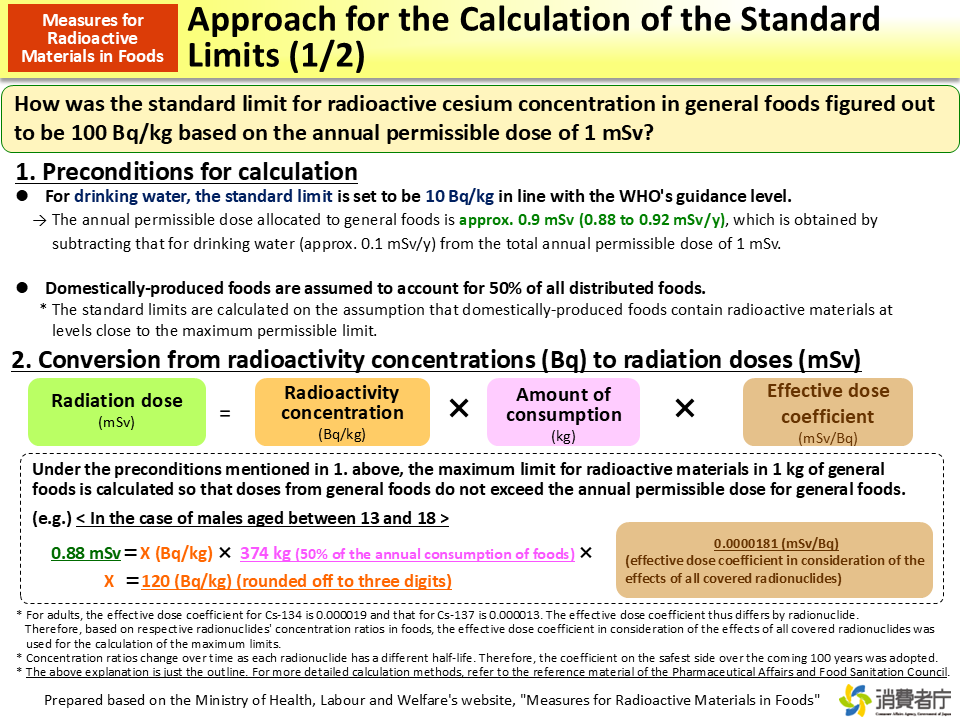BOOKLET to Provide Basic Information Regarding Health Effects of Radiation (2nd edition)

# Approach for the Calculation of the Standard Limits (1/2)This figure shows the approach for the calculation of the standard limits, explaining the relation between the annual dose limit (1 mSv) and the standard limit for radioactive cesium concentration in general foods (100 Bq/kg).

First, the annual permissible dose of 0.88 to 0.92 mSv is allocated to general foods by subtracting approx. 0.1 mSv permitted for drinking water from the total annual permissible dose of 1 mSv. Next, in consideration of the status of food self-sufficiency in Japan, it is assumed that 50% of all distributed foods (all of the domestically-produced foods) contains radioactive materials. Based on that assumption, in the case of males aged between 13 and 18, 374 kg of foods or 50% of the total annual consumption per person (approx. 748 kg) is supposed to be domestically produced. Additionally, the effective dose coefficient in consideration of the effects of all covered radionuclides (0.0000181 mSv/Bq) is to be used for calculation.

Then, the calculation formula is as follows.

0.88 mSv = (Radioactivity concentration: Bq/kg) × 374 kg × 0.0000181 (mSv/Bq)

(Radioactivity concentration: Bq/kg) = 120 Bq/kg

If concentrations of radioactive materials in general foods do not exceed 120 Bq/kg, the annual dose will remain within 0.88 mSv.

Therefore, the standard limit for general foods (100 Bq/kg), which is lower than 120 Bq/kg, is the value set on the safe side to guarantee safety.

• Included in this reference material on March 31, 2013
• Updated on March 31, 2019